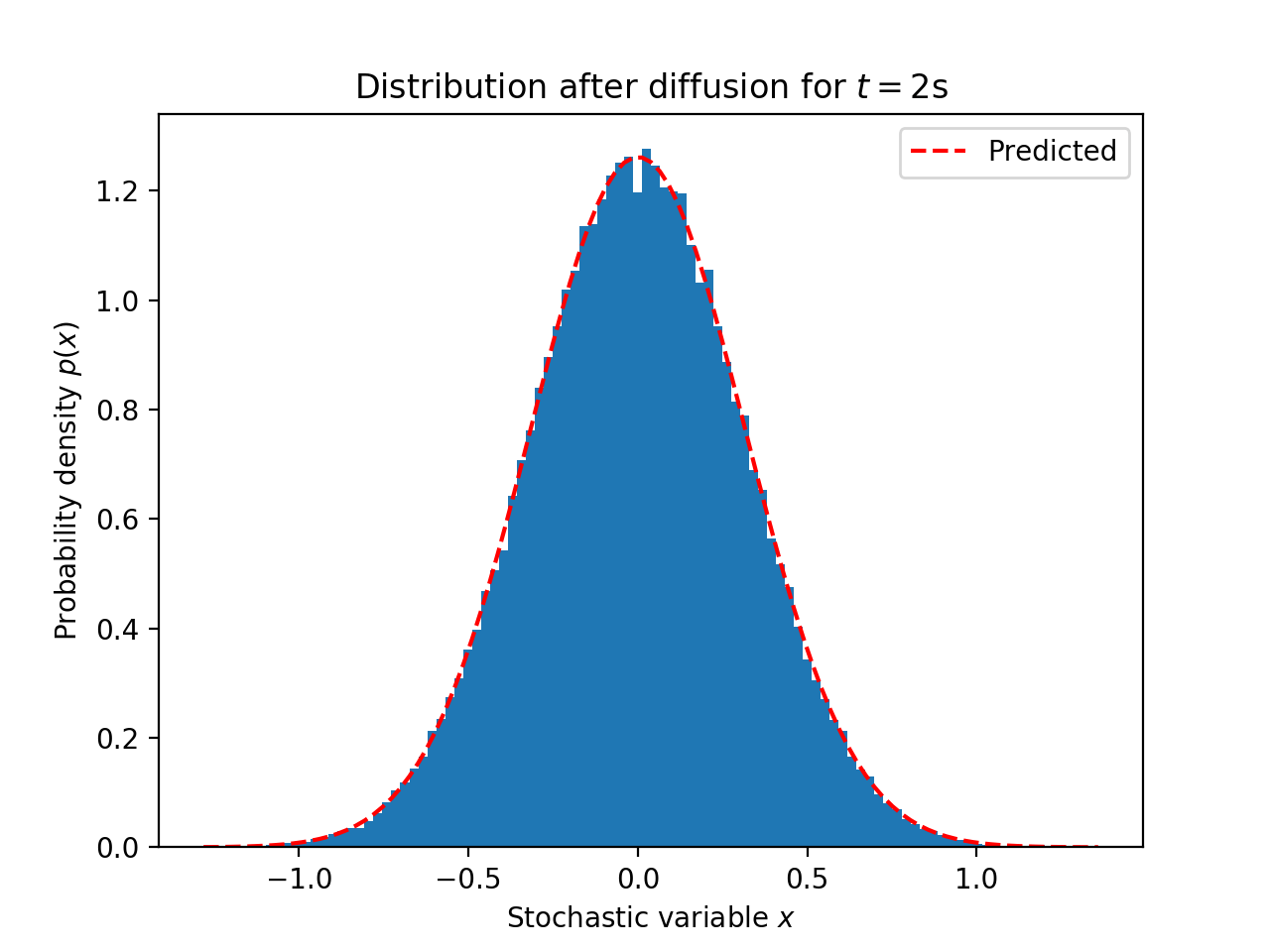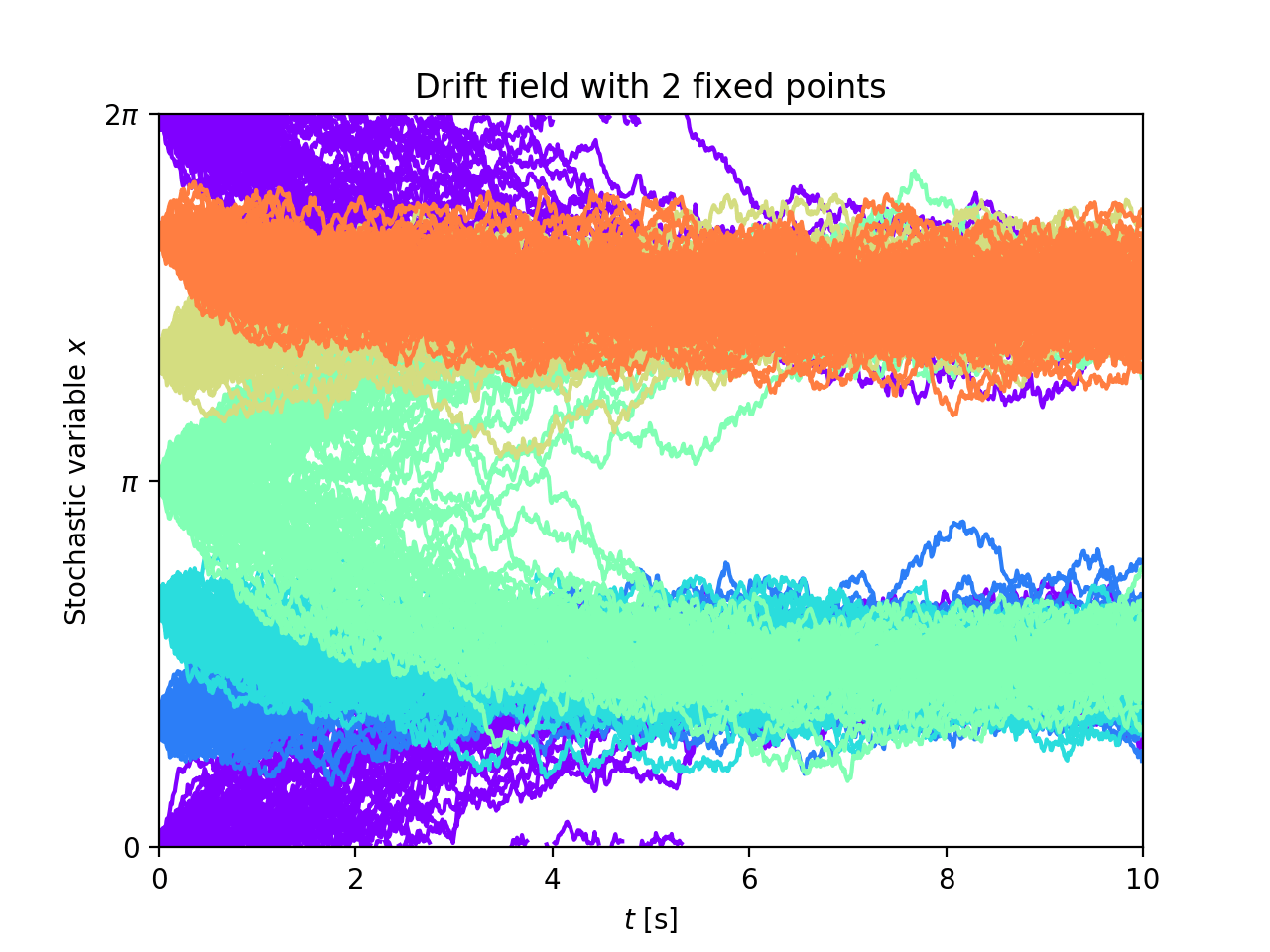This was published a while ago on Github.

Langevin integrator for SDEs with constant drift and diffusion on continuous intervals with circular boundary conditions.

CILES is written in Cython and uses GSL for interpolation of drift & diffusion fields, to be able to simulate continuous variables.

### Description

Given a discretized drift field A(x) and a (position dependent) diffusion coefficient B(x) this tool performs simple time-forward integration of the SDE:

```dx(t)/dt = A(x(t)) + sqrt(B(x(t))) * eta(t)
```

where eta(t) is a gaussian white noise term and x is a variable on an interval with circular boundaries (commonly 0 <= x < 2PI).

Both drift field A and diffusion B need to be arrays of the same dimension. They are internally interpolated (using`gsl_interp_cspline_periodic`) to provide continuous fields, which are then used in the forward integration.

Forward integration is performed with the Euler-Murayama scheme

```x(t+dt) = x(t) + dt * A(x(t)) + r * sqrt(dt * B(x(t)))
```

where r is a normally distributed random number with zero mean and unit variance.

### Installation

To install ciles in your Python distribution: – Clone repository – `python setup.py install` – To test (using `nosetests`): `nosetests`

You can also use ciles locally without installing: – Clone repository – `python setup.py build_ext --inplace`

### Example use

```from ciles.integrator import LangevinIntegrator as LI
import numpy as np

drift = np.zeros(100) # no drift field
diff = np.ones(100) # constant diffusion with 1 deg^2/s

dt = 1e-3 # 1 ms timestep
tmax = 1. # simulate until 1s

# initialize the integrator
li = LI(drift, diff, dt=dt, tmax=tmax)

# simulate a single trajectory
li.run(1)
out = li.out```

### More examples

Below are the plot results of the currently available examples from ciles.examples.

#### Final distributions after 2s diffusion

See the source#### Trajectories for drift-field with 2 fixed points

See the source